# Range S-S锁的获取规则

MSDN 对 Range 锁的规则有部分描述，但是言简意赅，以下我们会将各种情况分解开来，理清MSDN中涉及的或者未涉及的规则，这些规则适用于SQL Server 2000/2005/2008/2008 R2。关于MSDN的描述，请参见：http://technet.microsoft.com/zh-cn/library/ms191272(en-us,SQL.110).aspx。

CREATE TABLE [dbo].[MyTable](
[id] [int] IDENTITY(1,1) NOT NULL,
[index_column] [int] NOT NULL,
[data] [int] NOT NULL,
CONSTRAINT [PK_MyTable] PRIMARY KEY NONCLUSTERED
(
[id] ASC
)WITH (PAD_INDEX  = OFF, STATISTICS_NORECOMPUTE  = OFF, IGNORE_DUP_KEY = OFF, ALLOW_ROW_LOCKS  = ON, ALLOW_PAGE_LOCKS  = ON) ON [PRIMARY]
) ON [PRIMARY]

CREATE UNIQUE CLUSTERED INDEX [IX_MyTable] ON [dbo].[MyTable]
(
[index_column] ASC
)WITH (PAD_INDEX  = OFF, STATISTICS_NORECOMPUTE  = OFF, SORT_IN_TEMPDB = OFF, IGNORE_DUP_KEY = OFF, DROP_EXISTING = OFF, ONLINE = OFF, ALLOW_ROW_LOCKS  = ON, ALLOW_PAGE_LOCKS  = ON) ON [PRIMARY]

INSERT INTO [MyTable] ([index_column],[data]) VALUES (1, 1)
INSERT INTO [MyTable] ([index_column],[data]) VALUES (2, 2)
INSERT INTO [MyTable] ([index_column],[data]) VALUES (3, 3)
INSERT INTO [MyTable] ([index_column],[data]) VALUES (4, 4)
INSERT INTO [MyTable] ([index_column],[data]) VALUES (5, 5)
INSERT INTO [MyTable] ([index_column],[data]) VALUES (15, 6)
INSERT INTO [MyTable] ([index_column],[data]) VALUES (16, 7)
INSERT INTO [MyTable] ([index_column],[data]) VALUES (18, 8)
INSERT INTO [MyTable] ([index_column],[data]) VALUES (25, 9)
INSERT INTO [MyTable] ([index_column],[data]) VALUES (30, 10)

 id index_column data index hash row page 1 1 1 (8194443284a0) 78 2 2 2 (61a06abd401c) 78 3 3 3 (98ec012aa510) 78 4 4 4 (a0c936a3c965) 78 5 5 5 (59855d342c69) 78 6 15 6 (f1de2a205d4a) 78 7 16 7 (f07ed88b2b23) 78 8 18 8 (e9069d930a93) 78 9 25 9 (b81181109ebc) 78 10 30 10 (8034b699f2c9) 78

## 对于WHERE子句中的条件命中现有记录的情况

SELECT [data] FROM [MyTable] WHERE [index_column]=1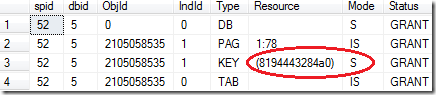SELECT [data] FROM [MyTable] WHERE [index_column]>=1 AND [index_column]<=4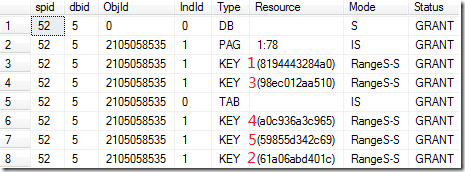SELECT [data] FROM [MyTable] WHERE [index_column]>=20 AND [index_column]<=40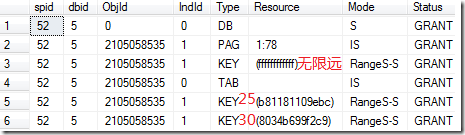SELECT [data] FROM [MyTable]

WHERE ([index_column]>=2 AND [index_column]<=4) OR ([index_column]>=10 AND [index_column]<=16) OR ([index_column]>=30 AND [index_column]<=40)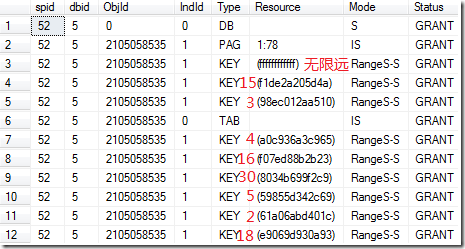## 对于WHERE子句中的条件不能命中任何记录的情况

SELECT [data] FROM [MyTable] WHERE [index_column]=6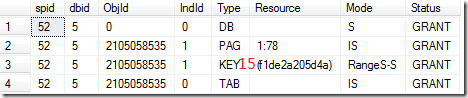SELECT [data] FROM [MyTable] WHERE [index_column]=31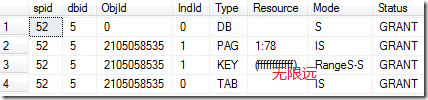SELECT [data] FROM [MyTable] WHERE [index_column]>=6 AND [index_column]<=10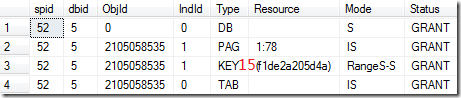SELECT [data] FROM [MyTable] WHERE [index_column]>30 AND [index_column]<40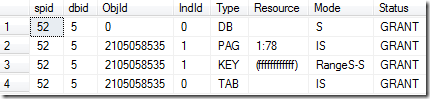posted @ 2011-10-21 01:49  TW－刘夏  阅读(3966)  评论(7编辑  收藏